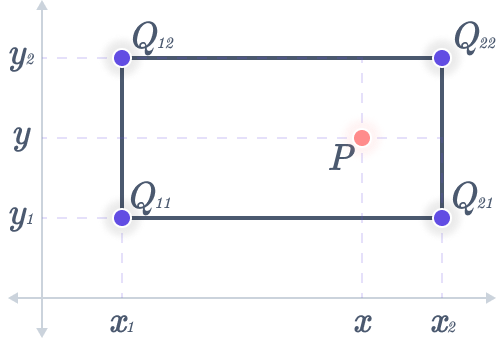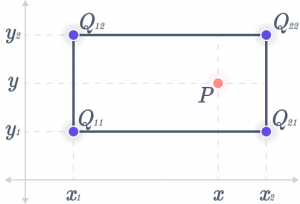# Bilinear Interpolation Calculator

A Bilinear interpolation calculator is a mathematical calculator configured to determine the location of a point provided by two coordinates. This calculator is helpful in practical situations when two coordinates and a point to bridge the gap must be known.Corner points coordinates

Corner points values

Interpolated point

## What is bilinear interpolation?

Bilinear interpolation is a common technique for two-dimensional interpolation on a rectangle. Values are estimated between known values using Bilinear interpolation. It is a method for figuring out where one point on a curve is in relation to the locations of the other two points.

## Bilinear Interpolation Formula

The following actions must be taken to find point P(x, y) using bilinear interpolation:

One linear interpolation along the y-axis, finding point P, and two linear interpolations along the x-axis, discovering intermediate points $$R_1$$ and $$R_2$$.

You can see that bilinear interpolation consists of one linear interpolation along the y-axis and two linear interpolations along the x-axis.The definition of Point $$R_1(x, y)$$ is

$$R_1(x, y) = \dfrac{Q_{11} (x_2 – x)}{(x_2 – x_1)} + \dfrac{Q_{21} (x – x_1)}{(x_2 – x_1)}$$

Point $$R_2(x, y)$$ is given as follows:

$$R_2(x, y) = \dfrac{Q_{12} (x_2 – x)}{(x_2 – x_1)} + \dfrac{Q_{22} (x – x_1)}{(x_2 – x_1)}$$

We define the interpolated point P(x, y) as follows:

$$P(x, y) = \dfrac{R_1 (y_2 – y)}{(y_2 – y_1)} + \dfrac{R_2 (y – y_1)}{(y_2 – y_1)}$$

## Why Do We Use Bilinear Interpolation?

The most often utilized applications of bilinear interpolation in engineering and science are as follows:

• Basic image processing and resampling methods are part of computer vision
• As a method to extract values from a specified set of data, embedded control systems

## FAQs

What is the Linear interpolation formula?

The linear interpolation formula is used to fit curves with linear polynomials. In essence, the interpolation approach generates new values for any function using the existing values.

The Linear Interpolation Formula is

$$y = y_1 + (\dfrac{x – x_1}{x_2 – x_1})(y_2 – y_1)$$

Where x is the known and y is the unknown value, x1 and y1 are the coordinates below the x value, and x2 and y2 are the coordinates above the x value.

What is the use of bilinear interpolation in MATLAB?

We can do image zooming using Bilinear Interpolation in MATLAB. Other software has an option of zooming an image too. For example in photoshop, you can zoom your image quite easily by clicking a button. We can do the same thing with MATLAB Bilinear Interpolation using code.